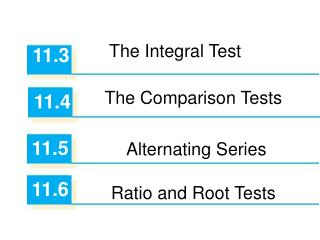# 11.3 - PowerPoint PPT PresentationDownload Presentation11.3

11.3Download Presentation## 11.3

- - - - - - - - - - - - - - - - - - - - - - - - - - - E N D - - - - - - - - - - - - - - - - - - - - - - - - - - -
##### Presentation Transcript

1. The Integral Test 11.3 • The Comparison Tests • 11.4 • 11.5 • Alternating Series • 11.6 • Ratio and Root Tests

2. Definition of Convergence for an infinite series: • Let be an infinite series of positive terms. • The series converges if and only if the sequence of partial sums, , converges. This means: Divergence Test: • If , the series diverges. • is divergent since • Example: The series • However, • does not imply convergence!

3. Geometric Series: The Geometric Series: converges for If the series converges, the sum of the series is: Example: The series with and converges . The sum of the series is 35.

4. p-Series: The Series: (called a p-series) converges for and diverges for Example: The series is convergent. The series is divergent.

5. Integral test: If f is a continuous, positive, decreasing function on with then the series converges if and only if the improper integral converges. Example: Try the series: Note: in general for a series of the form:

6. Comparison test: If the series and are two series with positive terms, then: • If is convergent and for all n, then converges. • If is divergent and for all n, then diverges. • (smaller than convergent is convergent) • (bigger than divergent is divergent) Examples: which is a divergent harmonic series. Since the original series is larger by comparison, it is divergent. which is a convergent p-series. Since the original series is smaller by comparison, it is convergent.

7. Limit Comparison test: If the the series and are two series with positive terms, and if where then either both series converge or both series diverge. Useful trick: To obtain a series for comparison, omit lower order terms in the numerator and the denominator and then simplify. Examples: For the series compare to which is a convergent p-series. For the series compare to which is a divergent geometric series.

8. Alternating Series test: If the alternating series satisfies: and then the series converges. Definition: Absolute convergence means that the series converges without alternating (all signs and terms are positive). Example: The series is convergent but not absolutely convergent. Alternating p-series converges for p > 0.   Example: The series and the Alternating Harmonic series are convergent.

9. Ratio test: • If then the series converges; • If the series diverges. • Otherwise, you must use a different test for convergence. If this limit is 1, the test is inconclusive and a different test is required. Specifically, the Ratio Test does not work for p-series. Example:

10. 11.7 • Strategy for Testing Series

11. Summary: • Apply the following steps when testing for convergence: • Does the nth term approach zero as n approaches infinity? If not, the Divergence Test implies the series diverges. • Is the series one of the special types - geometric, telescoping, p-series, alternating series? • Can the integral test, ratio test, or root test be applied? • Can the series be compared in a useful way to one of the special types?

12. Summaryof all tests:

13. Example • Determine whether the series converges or diverges using the Integral Test. • Solution: Integral Test: Since this improper integral is divergent, the series  (ln n)/n is also divergent by the Integral Test.

14. More examples

15. Example 1 • Since as an ≠ as n  , we should use the Test for Divergence.

16. Example 2 Since an is an algebraic function of, we compare the given series with a p-series. The comparison series for the Limit Comparison Test is where

17. Example 4 Since the series is alternating, we use the Alternating Series Test.

18. Example 5 • Since the series involves k!, we use the Ratio Test.

19. Example 6 Since the series is closely related to the geometric series , we use the Comparison Test.